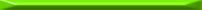Tags SICP

Some important quotes from the book:

We began section 1.3 with the observation that compound procedures are a crucial abstraction mechanism, because they permit us to express general methods of computing as explicit elements in our programming language. Now we’ve seen how higher-order procedures permit us to manipulate these general methods to create further abstractions.

[...] In general, programming languages impose restrictions on the ways in which computational elements can be manipulated. Elements with the fewest restrictions are said to have first-class status. Some of the ``rights and privileges’’ of first-class elements are: (1) They may be named by variables; (2) They may be passed as arguments to procedures; (3) They may be returned as the results of procedures; (4) They may be included in data structures; Lisp, unlike other common programming languages, awards procedures full first-class status. This poses challenges for efficient implementation, but the resulting gain in expressive power is enormous.

Here is the code for `newtons-method` in CL. Note that this time I’ll follow Lisp’s convention of signifying the names of global variables by asterisks:

```(defvar *tolerance* 0.00001)

(defun fixed-point (f first-guess)
(labels (
(close-enough? (v1 v2)
(< (abs (- v1 v2)) *tolerance*))
(try (guess)
(let ((next (funcall f guess)))
(if (close-enough? guess next)
next
(try next)))))

(try first-guess)))

(defvar *dx* 0.00001)

(defun deriv (g)
(lambda (x)
(/  (-  (funcall g (+ x *dx*))
(funcall g x))
*dx*)))

(defun newton-transform (g)
(lambda (x)
(- x  (/ (funcall g x)
(funcall (deriv g) x)))))

(defun newtons-method (g guess)
(fixed-point (newton-transform g) guess))
```

Note again that CL’s semantics of handling functions makes the code a little more cumbersome than the Scheme code of the authors. While anonymous functions defined by `lambda` can be passed as arguments where functions are expected (for example the second argument in the call to `fixed-point` in `newtons-method`), when applied to arguments explicitly, a `funcall` must be used (for example the application of `(deriv g)` in the last line of `newton-transform`).

#### Exercise 1.40

This is just an exercise in building functions from arguments and returning them.

```(defun cubic (a b c)
(lambda (x)
(+  (cube x)
(* a (square x))
(* b x)
c)))
```

Note that `cubic` is a function builder – each time it’s called with some arguments, it creates a new function and returns is.

#### Exercise 1.41

Again, this is a function builder. It is even more interesting, because its argument is a function and not numbers. So `double` takes a function as an argument and returns a function – it’s almost a full case study of higher-order functions by itself!

```(defun double (f)
(lambda (x)
(funcall f (funcall f x))))

(print (funcall (double #'1+) 1))
=>
3
```

What does `(double double)` do ? Applies f twice to its argument, when f is a function that applies some function twice to its argument. Therefore, it applies the function it receives 4 times. `((double (double double))` applies the function it receives 4*4 = 16 times. Therefore:

```(print  (funcall
(funcall
(double (double #'double))
#'1+)
5))
=>
21
```

#### Exercise 1.42

This is very similar to the previous exercise:

```(defun compose (f g)
(lambda (x)
(funcall f (funcall g x))))
```

#### Exercise 1.43

```(defun repeated (f n)
(if (= n 0)
#'identity
(compose f (repeated f (1- n)))))
```

This is a process that “accumulates” applications of `f` on itself. Note the stop condition – `identity` is returned for `n = 0`. This means that for `n = 1`, `f` is composed with `identity`, and the result is just applying `f` once, which is what we need.

#### Exercise 1.44

```(defvar *dx* 0.00001)

(defun smooth (f)
(lambda (x)
(/ (+ (funcall f (- x *dx*))
(funcall f x)
(funcall f (+ x *dx*)))
3)))

(defun n-fold-smooth (f n)
(funcall (repeated #'smooth n) f))
```

#### Exercise 1.45

First, recall the code of section 1.3.3 and the solutions to its exercises. Here is the `dampen-sqrt` function again:

```(defvar *tolerance* 0.00001)

(defun fixed-point (f first-guess)
(labels (
(close-enough? (v1 v2)
(< (abs (- v1 v2)) *tolerance*))
(try (guess)
(let ((next (funcall f guess)))
(if (close-enough? guess next)
next
(try next)))))

(try first-guess)))

(defun average (a b)
(/ (+ a b) 2))

(defun dampen-sqrt (x)
(fixed-point
(lambda (y)
(average y (/ x y)))
1.0))
```

Now, let’s implement a more general dampening function for any root of x:

```(defun dampen-root (x n)
(fixed-point
(lambda (y)
(average y (/ x (expt y (1- n)))))
1.0))
```

`n` will be 2 for the square root, 3 for the cube root, 4 for the 4th root and so on. Using this function we can try computing various roots:

```(print (dampen-root 2 2))
=> 1.4142
(print (dampen-root 2 3))
=> 1.2599
(print (dampen-root 2 4))
=> *** - Program stack overflow. RESET
```

Indeed, the simple 1-step dampening doesn’t work for the 4th root – the computation doesn’t converge. So let’s follow the authors’ advice and implement a repeated dampening1 version:

```(defun repeated-dampen-root (x nroot nrepeat)
(fixed-point-of-transform
(lambda (y) (average y (/ x (expt y (1- nroot)))))
(repeated #'average-damp nrepeat)
1.0))

(print (repeated-dampen-root 2 4 2))
=> 1.89
```

This is better.

#### Exercise 1.46

```(defun iterative-improve (good-enough? improve)
(lambda (first-guess)
(labels (
(improve-iter (guess)
(let ((improved-guess (funcall improve guess)))
(if (funcall good-enough? guess improved-guess)
improved-guess
(improve-iter improved-guess)))))

(improve-iter first-guess))))

(defun improved-sqrt (num)
(funcall  (iterative-improve
(lambda (x y)
(let ((ratio (/ x y)))
(and (< ratio 1.001) (> ratio 0.999))))
(lambda (guess)
(average guess (/ num guess))))
1.0))

(defvar *tolerance* 0.00001)

(defun improved-fixed-point (f first-guess)
(funcall  (iterative-improve
(lambda (x y)
(< (abs (- x y)) *tolerance*))
(lambda (guess)
(funcall f guess)))
first-guess))
```1 Thanks to Sean who suggested a bug fix in this function (see Comments to this post).